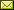## Jacobians and Gradients for Cartesian Differential Categories

 Jean-Simon Pacaud Lemay (Mount Allison University)

 Cartesian differential categories come equipped with a differential combinator that formalizes the directional derivative from multivariable calculus. Cartesian differential categories provide a categorical semantics of the differential lambda-calculus and have also found applications in causal computation, incremental computation, game theory, differentiable programming, and machine learning. There has recently been a desire to provide a (coordinate-free) characterization of Jacobians and gradients in Cartesian differential categories. One's first attempt might be to consider Cartesian differential categories which are Cartesian closed, such as models of the differential lambda-calculus, and then take the curry of the derivative. Unfortunately, this approach excludes numerous important examples of Cartesian differential categories such as the category of real smooth functions. In this paper, we introduce linearly closed Cartesian differential categories, which are Cartesian differential categories that have an internal hom of linear maps, a bilinear evaluation map, and the ability to curry maps which are linear in their second argument. As such, the Jacobian of a map is defined as the curry of its derivative. Many well-known examples of Cartesian differential categories are linearly closed, such as, in particular, the category of real smooth functions. We also explain how a Cartesian closed differential category is linearly closed if and only if a certain linear idempotent on the internal hom splits. To define the gradient of a map, one must be able to define the transpose of the Jacobian, which can be done in a Cartesian reverse differential category. Thus, we define the gradient of a map to be the curry of its reverse derivative and show this equals the transpose of its Jacobian. We also explain how a linearly closed Cartesian reverse differential category is precisely a linearly closed Cartesian differential category with an appropriate notion of transpose.

In Kohei Kishida: Proceedings of the Fourth International Conference on Applied Category Theory (ACT 2021), Cambridge, United Kingdom, 12-16th July 2021, Electronic Proceedings in Theoretical Computer Science 372, pp. 29–42.
Published: 3rd November 2022.

 ArXived at: http://dx.doi.org/10.4204/EPTCS.372.3 bibtex PDF
References in reconstructed bibtex, XML and HTML format (approximated).
 Comments and questions to:eptcs@eptcs.org For website issues:webmaster@eptcs.org